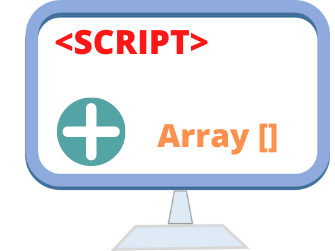# How to create array and add elementWe can create arrays in JavaScript in different ways. If we are processing a form then elements of the form can be managed as part of an array. We will learn some different points on use of arrays and before that we will learn some basics of JavaScript array.

How to create arrays in JavaScript?

• ### Creating and adding elements to Array

We can declare an array like this
``var scripts = new Array();``
We can add elements to this array like this
``````
scripts = "PHP";
scripts = "ASP";
scripts = "JavaScript";
scripts = "HTML";
``````
Now our array scrips has 4 elements inside it and we can print or access them by using their index number. Note that index number starts from 0. To get the third element of the array we have to use the index number 2 . Here is the way to get the third element of an array.
``document.write(scripts);``
We also can create an array like this
``````var no_array = new Array(21, 22, 23, 24, 25); // Array has 5 elements
var my_array=['4th']; // array with single element``````

## Crating Array from a String

More practical requirement will be crating array from a string. You can read more on this on our tutorial on split.## Subscribe

* indicates required
Subscribe to plus2netplus2net.com